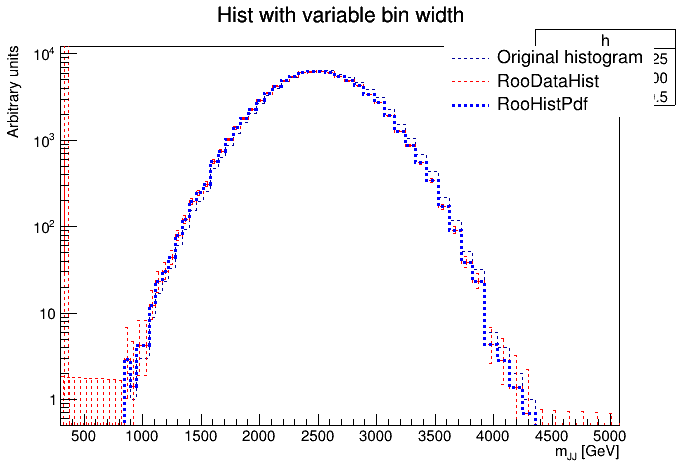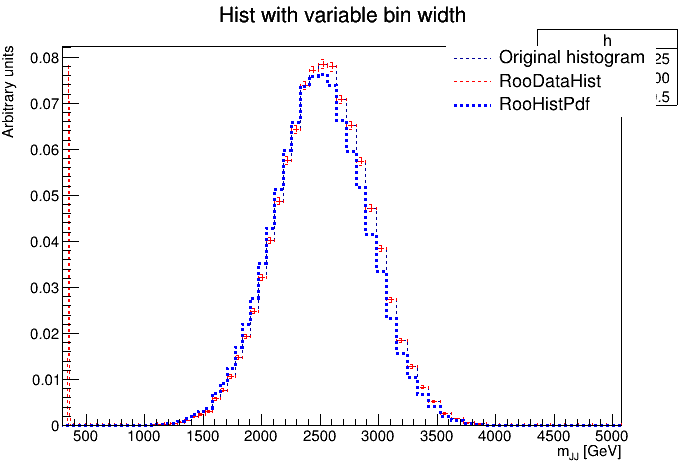# RooHistPdf and variable bin width

Dear Roofit experts,

I’m trying to convert an histogram created with variable bin widths into a RooHistPdf, but the two curves don’t seem to match, as you can see in the plot below:I also attached the code that allows to create that curves:variable_bin_test.C (1.6 KB)
Would it be possible to tell me what I should change in my code?

Many thanks.
Best regards,
Nathan

If I remember correctly, RooFit is dividing by the bin width. This makes sense for a PDF, since it’s a probability density. It’s hard to see from the log plot, but can you check that this is indeed the difference?

Dear Stephan,

Here is the plot without the log scale:I also normalized the original histogram and now it seems that it corresponds to the RooDataHist whereas it was not case in the previous plot.
Do you mean that Roofit divide each bin by the bin width? Because the bins are between ~50 and ~100 GeV wide, so we would see a bigger offset between the histogram and the RooHistPdf, no?
If I misunderstood, is there any way to deactivate this feature and make the histogram and the RooHistPdf to match?

Thank you again.
Best,
Nathan

Yes, RooFit divides by the bin width, because a PDF otherwise isn’t a PDF (it’s needed for the density as mentioned above).
Can it be that the bin width changes quite a bit near 2400, where the bins jump from “all too high” to “all too low” in comparison to the data histogram? If that’s the case, the difference we see is because of the bin width correction.

If I’m not mistaken, there is currently no option to switch this off, but there might be an option to tell the RooDataHist to apply the same correction. I haven’t found it yet, but I will give it another look.

It’s a bit hacky, because you have to configure the plot options manually, but this is how you can do it:

`````` 32   RooAbsData::PlotOpt opts;
33   opts.bins = &mjjbin;
34   opts.correctForBinWidth = true;
35   opts.etype = RooAbsData::None;
36   hdata.plotOn(frameData, opts);
``````

The corresponding function is here:
https://root.cern.ch/doc/master/classRooDataHist.html#acce2f981ef2374868e9c4d05f55aab12
and the `PlotOpt` here:
https://root.cern.ch/doc/master/structRooAbsData_1_1PlotOpt.html

## Note:

This only works with the error types `None` and `SumW2`, since the default `Poisson` errors don’t work for non-integer bin counts. If the bin width correction is in effect, the bin counts are most likely not integers.

Dear Stephan, dear experts,

I have a related question. For what I understood, both RooDataHist and related RooHistPdf obtained from a TH1 are divided by the bin widths when created.
This is ok for me, I checked that explicitly with a minimal scripts which creates two simple TH1, one in variable v1 (3 uniform bins), and one in variable v2 (2 non-uniform bins), as seen in the attached plots v1_1D and v2_1D (the RooDataHist are in black, the RooHistPdf in red).
I have a problem when I repeat the exercise with a TH2 in (v1,v2), with same binnings, and bin contents set in a way that its projections are equal to the TH1 created in the previous step.
The plotted RooDataHist gives me something expected (see canvas v1_2D and v2_2D), but I see something weird for the RooHistPdf in the projection on v2 (v2_2D).
Am I wrong in expecting something exactly equal to the v1_1D, v2_1D plots, or is there something wrong somewhere?

Thanks a lot
Federico

test2.C (1.7 KB)

Hey @fbetti,

at first sight, you might be seeing the same as `nathanL`: Unless you ask for the data to be normalised by bin width, it plots the bare counts. In examples 1-3, the bin widths seem to be identical. In example 4, they are not. Did you try switching on the bin width normalisation also for data as discussed above?

By the way:
To nicely plot on one canvas, you may also use

``````canvas->Divide(2,2);
canvas->cd(1);
...
canvas->cd(2);
...
``````

Thanks for the prompt answer @StephanH.

Unless you ask for the data to be normalised by bin width, it plots the bare counts

Actually, I see the opposite: the TH1 in v2 has 45 entries in bin 1 and 90 in bin 2, but the second bin is twice wider than the first, so the corresponding RooDataHist, when plotted, show them correctly, and the same happen for the RooHistPdf.
This is not a problem for me, I am fine with showing data divided by the bin width.
What concerns me is that I can not explain what happens when I move to two dimensions (bottom plots). Everything is fine in v1_2D (uniform binning), but I can’t understand why the RooHistPdf is different in the projection on v2 (v2_2D plot). Even trying to switch on bin width normalisation as you suggest (but isn’t it already done by RooFit?) I get the very same result. I attach here the macro. test3.C (2.5 KB)

cheers,
Federico

The difference between those two cases is indeed that a projection integral is running. In fact, it integrates the PDF twice, once over `(v1, v2)` to find the total number of events. Here, it’s just retrieving the total number of counts in the data hist. This is needed for the global normalisation, and I assume that’s correct.
The second time, the PDF is integrated over `v1` to project it onto the `v2` axis. For the projection integral, it’s actually dividing each bin by its bin width. I quickly checked if multiplying or not dividing at all yields better results, but in both cases, the plot is completely off.
If you use

``````pdf.forceNumInt(true);
``````

you get what you expect, though. This points to a problem in the (analytic) projection function.

I haven’t really figured out yet what to do differently to project this thing correctly. Any idea on your side?

Yes, I confirm that

``````pdf.forceNumInt(true);
``````

works.

Unfortunately no, I am definitely not enough expert to understand in detail what is actually happening.
But if this issue appears only when the plot is performed, I think the forceNumInt is a sufficient workaround. My concern is that something bad could happen for example during the fit. I tested a dumb fit (adding a flat background) and I get what I expect, even if the plot looks bad. Repeating the forceNumInt give very slightly different results (with a good plot), but I think this is ok, since in this way numerical integration is performed and numerical difference could enter the game, right?
As you said, there is still something tricky in the analytic projection, which I can not understand.

During the fit, unless you project out a 1D distribution from the histogram, no projection integrals are needed. Therefore, the relevant part of the code is not used.

Right, thank you for the clarification.

Dear Stephan,

thank you very much and sorry for the late answer.
So, if I understand correctly, we can force the RooDataHist and the RooHistPdf to match, but there is no way to match the original histogram and the RooHistPdf, right?

Best,
Nathan

Maybe there is, but I don’t know the details. There is an option to import a density when you create a RooDataHist. See here: